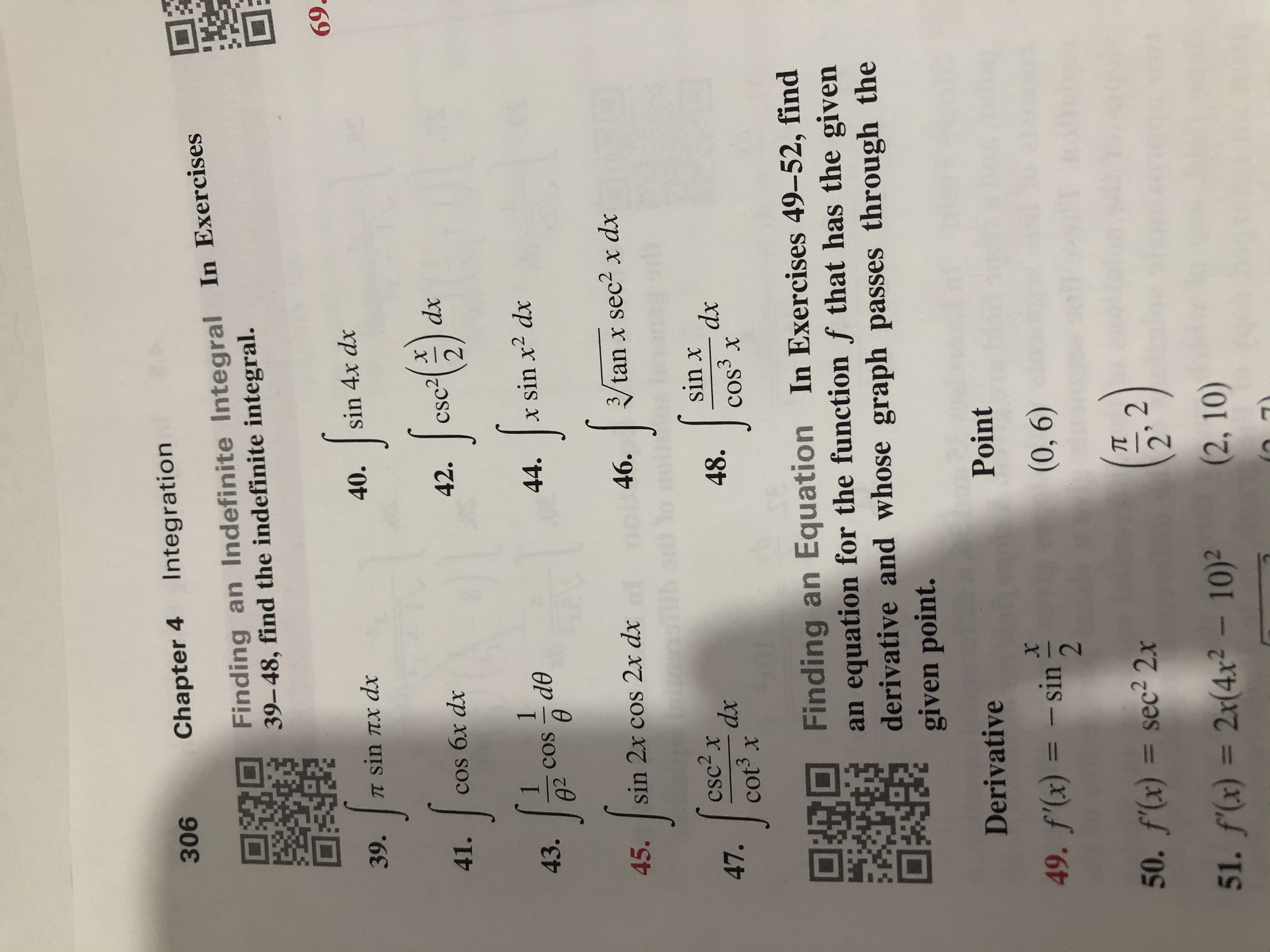# IntegrationChapter 4306Finding an Indefinite Integral In Exercises39-48, find the indefinite integral.69sin 4x dx40.TT sin Tx dx39.dxcsc242.41.cos бx dxx sin x2 dx44.de43.COS023tan x sec2 x dx46.sin 2x cos 2x dx45.sin xdxcos3 xcsc2xdxcot3 x48.47.Finding an Equation In Exercises 49-52, findan equation for the function f that has the givenderivative and whose graph passes through thegiven point.DerivativePoint(0, 6)49. f(x)- sin11=50. f'(x) = sec2 2.x251. f(x) = 2x(4x2- 10)2(2, 10)

Question
1 views

39help_outlineImage TranscriptioncloseIntegration Chapter 4 306 Finding an Indefinite Integral In Exercises 39-48, find the indefinite integral. 69 sin 4x dx 40. TT sin Tx dx 39. dx csc2 42. 41. cos бx dx x sin x2 dx 44. de 43. COS 02 3 tan x sec2 x dx 46. sin 2x cos 2x dx 45. sin x dx cos3 x csc2x dx cot3 x 48. 47. Finding an Equation In Exercises 49-52, find an equation for the function f that has the given derivative and whose graph passes through the given point. Derivative Point (0, 6) 49. f(x) - sin 11 = 50. f'(x) = sec2 2.x 2 51. f(x) = 2x(4x2- 10)2 (2, 10) fullscreen
check_circle

Step 1

Given integral is, &...

### Want to see the full answer?

See Solution

#### Want to see this answer and more?

Solutions are written by subject experts who are available 24/7. Questions are typically answered within 1 hour.*

See Solution
*Response times may vary by subject and question.
Tagged in

### Integration